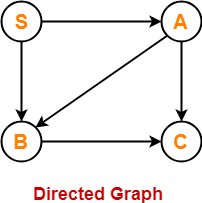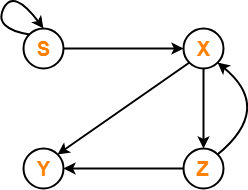Algorithm To Decide Whether CFL Is Finite

Context Free Language-

Before you go through this article, make sure that you have gone through the previous article on Context Free Language.

We have discussed-

• Context free language is generated using a context free grammar.
• Each context free language is accepted by a Pushdown automaton.

Algorithm To Decide Whether CFL Is Finite Or Not-

For a given CFG, there exists an algorithm to decide whether its language is finite or not.

Step-01:

Reduce the given grammar completely by-

• Eliminating ∈ productions
• Eliminating unit productions
• Eliminating useless productions

Also Watch- How To Reduce Grammar?

Step-02:

• Draw a directed graph whose nodes are variables of the given grammar.
• There exists an edge from node A to node B if there exists a production of the form A → αBβ.

Now, following 2 cases are possible-

Case-01:

• Directed graph contains a cycle.
• In this case, language of the given grammar is infinite.

Case-02:

• Directed graph does not contain any cycle.
• In this case, language of the given grammar is finite.

Also Read- Algorithm To Decide Whether CFL Is Empty

Problem-01:

Check whether language of the following grammar is finite or not-

S → AB / a

A → BC / b

B → CC / c

Solution-

Step-01:

The given grammar is already completely reduced.

Step-02:

We will draw a directed graph whose nodes will be S , A , B , C.

Now,

• Due to the production S → AB, directed graph will have edges S → A and S → B.
• Due to the production A → BC, directed graph will have edges A → B and A → C.
• Due to the production B → CC, directed graph will have edge B → C.

The required directed graph is-Clearly,

• The directed graph does not contain any cycle.
• Therefore, language of the given grammar is finite.

Problem-02:

Check whether language of the following grammar is finite or not-

S → XS / b

X → YZ

Y → ab

Z → XY

Solution-

Step-01:

The given grammar is already completely reduced.

Step-02:

We will draw a directed graph whose nodes will be S , X , Y , Z.

Now,

• Due to the production S → XS / b, directed graph will have edges S → X and S → S.
• Due to the production X → YZ, directed graph will have edges X → Y and X → Z.
• Due to the production Z → XY, directed graph will have edges Z → X and Z → Y.

The required directed graph is-Clearly,

• The directed graph contain cycles.
• Therefore, language of the given grammar is infinite.

Next Article- CYK Algorithm

Get more notes and other study material of Theory of Automata and Computation.

Watch video lectures by visiting our YouTube channel LearnVidFun.

SummaryArticle Name
Algorithm To Decide Whether CFL Is Finite
Description
Decision Properties of Context Free Languages and Decision Algorithms for Context Free Languages- Algorithm to decide whether a Context Free Language is finite or not.
Author
Publisher Name
Gate Vidyalay
Publisher Logo